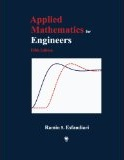## Inside: Applied Mathematics for Engineers, Fifth EditionApplied Mathematics for Engineers, Fifth Edition

# Preface

The principal goal of this book is to provide the reader with a comprehensive knowledge of fundamental concepts and methods of applied mathematics used in different engineering disciplines. Each topic is covered in great detail, followed by several meticulously worked-out examples, as well as a problem set containing a large number of additional related exercises. Engineering applications are stressed and integrated throughout the book, and consistent with that notion, derivations of the mathematical models of many physical systems are included to familiarize the reader with the basic laws and proper engineering terminology. The fourth edition of the book (2007) has been entirely revamped to produce this latest edition through the addition of several topics and removal of a few others.

The book is essentially divided into three main parts. The first part covers complex analysis (Chapter 1), ordinary and partial differential equations (Chapters 2–4 and 7), Laplace transformation (Chapter 5), and Fourier analysis (Chapter 6).

In the second part, a detailed coverage of matrix analysis (Chapter 8) and matrix eigenvalue problem (Chapter 9) is presented. Several applications in engineering vibrations are included for a better understanding of the effectiveness of matrix computations in determining systems’ natural frequencies and mode shapes.

The third part of the book is completely devoted to numerical methods with applications in different engineering disciplines. The powerful software MATLAB® is introduced in Chapter 10 and is used to perform symbolic, graphical, and numerical tasks. Chapter 11 introduces techniques for solving equations and systems. Chapter 12 discusses curve fitting and interpolation techniques, while Chapter 13 covers numerical differentiation and integration methods. Chapter 14 presents numerical methods for solving initial- and boundary-value problems. Chapter 15 introduces methods to approximate eigenvalues of a matrix. Numerical solution of partial differential equations is covered in Chapter 16.

An ample part of the material in this book has been rigorously class tested over the past several years, and the valuable remarks and suggestions made by students and professors who used the earlier editions have greatly contributed to making this new edition as complete and user-friendly as possible.

# Pedagogy of the Book

The book is written in a user-friendly manner to make the material easy to understand by the reader. In Chapters 1 through 9, each topic is accompanied by at least one fully worked-out example and a large number of exercises in the problem sets. In Chapters 10 through 16, the following format is utilized:

• Each method is accompanied by a MATLAB user-defined function or a script file that uses the method to perform a specific task
• This is followed by at least one thoroughly worked-out example showing all details
• The results are then confirmed through the execution of the user-defined function or the script file
• Whenever possible, MATLAB built-in functions are executed for re-confirmation of the results
• Plots are regularly generated to shed light on the accuracy and significance of the numerical results

# Exercises (Chapters 10–16)

A large set of problems/projects with different levels of difficulty is included at the end of each chapter and can be worked out either using a:

• hand calculator, or
• MATLAB

In many instances, the reader is asked to prepare a user-defined function or a script file that implements a specific technique, which often require revisions to those already presented in the chapter.